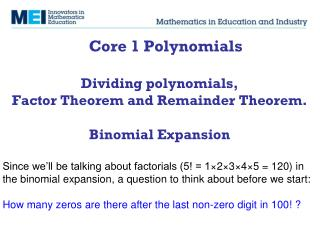DownloadDownload PresentationFactor Theorem Remainder Theorem

# Factor Theorem Remainder Theorem

Télécharger la présentation## Factor Theorem Remainder Theorem

- - - - - - - - - - - - - - - - - - - - - - - - - - - E N D - - - - - - - - - - - - - - - - - - - - - - - - - - -
##### Presentation Transcript

1. Core 1 PolynomialsDividing polynomials, Factor Theorem and Remainder Theorem.Binomial Expansion Since we’ll be talking about factorials (5! = 1×2×3×4×5 = 120) in the binomial expansion, a question to think about before we start: How many zeros are there after the last non-zero digit in 100! ?

2. Polynomial division Factor Theorem Remainder Theorem

3. Polynomial multiplication

4. Polynomial division

5. Polynomial division

6. Two to try

7. Polynomial division Factor Theorem Remainder Theorem

8. Factor Theorem If (x-a) is a factor of f(x), then f(a)=0 and x=a is a root of the equation f(x)=0. Conversely, if f(a)=0 then (x-a) is a factor of f(x).

9. Remainder Theorem For a polynomial f(x), f(a) is the remainder when f(x) is divided by (x-a).

10. MEI Core 1, June 10, Qn 6, 5 marks MEI Core 1, June 09, Qn 3, 3 marks

11. Binomial Expansion

12. Magic? 1 1 1 1 2 1 1 3 3 1 1 4 6 4 1

13. Why Pascal’s triangle gives the binomial coefficients

14. The problem with relying on Pascal’s triangle How would you find the coefficient of x7 in the expansion of

15. Pascal’s Triangle isn’t really about adding numbers – it’s about choosing. 1 1 1 1 2 1 1 3 3 1 1 4 6 4 1 1 5 10 10 5 1

16. Binomial Expansion

17. MEI Core 1, Jan 10, Qn 8, 4 marks

18. MEI Core 1, June 09, Qn 5, 4 marks

19. MEI Core 1, Jan 08, Qn 7, 4 marks

20. Two much more challenging questions involving nCr • How many anagrams of ANAGRAM do not contain adjacent As? • How many ways are there of writing 20 as a sum of exactly 4 positive integers where order matters? (3+8+8+1 is different from 1+3+8+8)